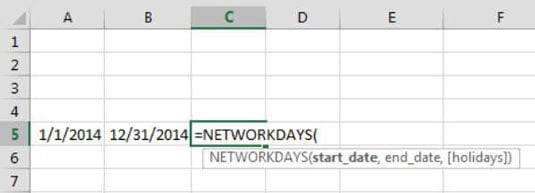##### Excel 2019 All-in-One For DummiesMost of the functions found in Excel require some input or information in order to calculate correctly. For example, to use the AVERAGE function, you need to give it a range of numbers to average.

`=AVERAGE(A1:A100)`

Any input you give to a function is called an argument.

The basic construct of a function is:

Function_Name(argument1, argument2,…)

To use a function, you enter its name, open parenthesis, the needed arguments, and then the close parenthesis. The number of arguments needed varies from function to function.

## Using functions with no arguments

Some functions, such as the NOW() function, don’t require any arguments. To get the current date and time, you can simply enter a formula like this:

`=NOW()`

Note that even though no arguments are required, you still need to include the open and close parentheses.

## Using functions with one or more required arguments

Some functions require one or more arguments. The LARGE function, for instance, returns the nth largest number in a range of cells. This function requires two arguments: a cell reference to a range of numeric values and a rank number. To get the third largest value in range A1 through A100, you can enter:

`=LARGE(A1:A100,3)`

Note that each argument is separated by a comma. This is true regardless of how many arguments you enter. Each argument must be separated by a comma.

## Using functions with both required and optional arguments

Many Excel functions, such as the NETWORKDAYS function, allow for optional arguments in addition to the required arguments. The NETWORKDAYS function returns the number of workdays (days excluding weekends) between a given start date and end data.

To use the NETWORKDAYS function, you need to provide, at minimum, the start and end dates. These are the required arguments.

The following formula gives you the answer 260, meaning that there are 260 workdays between January 1, 2014, and December 31, 2014:

`=NETWORKDAYS("1/1/2014", "12/31/2014")`

The NETWORKDAYS function also allows for an optional argument that lets you pass a range containing a list of holiday dates. The function treats each date in the optional range as a nonworkday, effectively returning a different result (255 workdays between January 1, 2014, and December 31, 2014, taking into account holiday dates).

`=NETWORKDAYS("1/1/2014", "12/31/2014", A1:A5)`

Don’t be too concerned with completely understanding the NETWORKDAYS function. The take-away here is that when a function has required and optional arguments, you can elect to use the function with just the required arguments, or you can take advantage of the function’s additional utility by providing the optional arguments.

## Finding out which arguments are needed for a given function

An easy way to discover the arguments needed for a given function is to begin typing that function into a cell. Click a cell, enter the equal sign, enter the function name, and then enter an open parenthesis.

Recognizing that you are entering a function, Excel activates a tooltip that shows you all the arguments for the function. Any argument that is shown in brackets ([ ]) is an optional argument. All others shown without the brackets are required arguments.